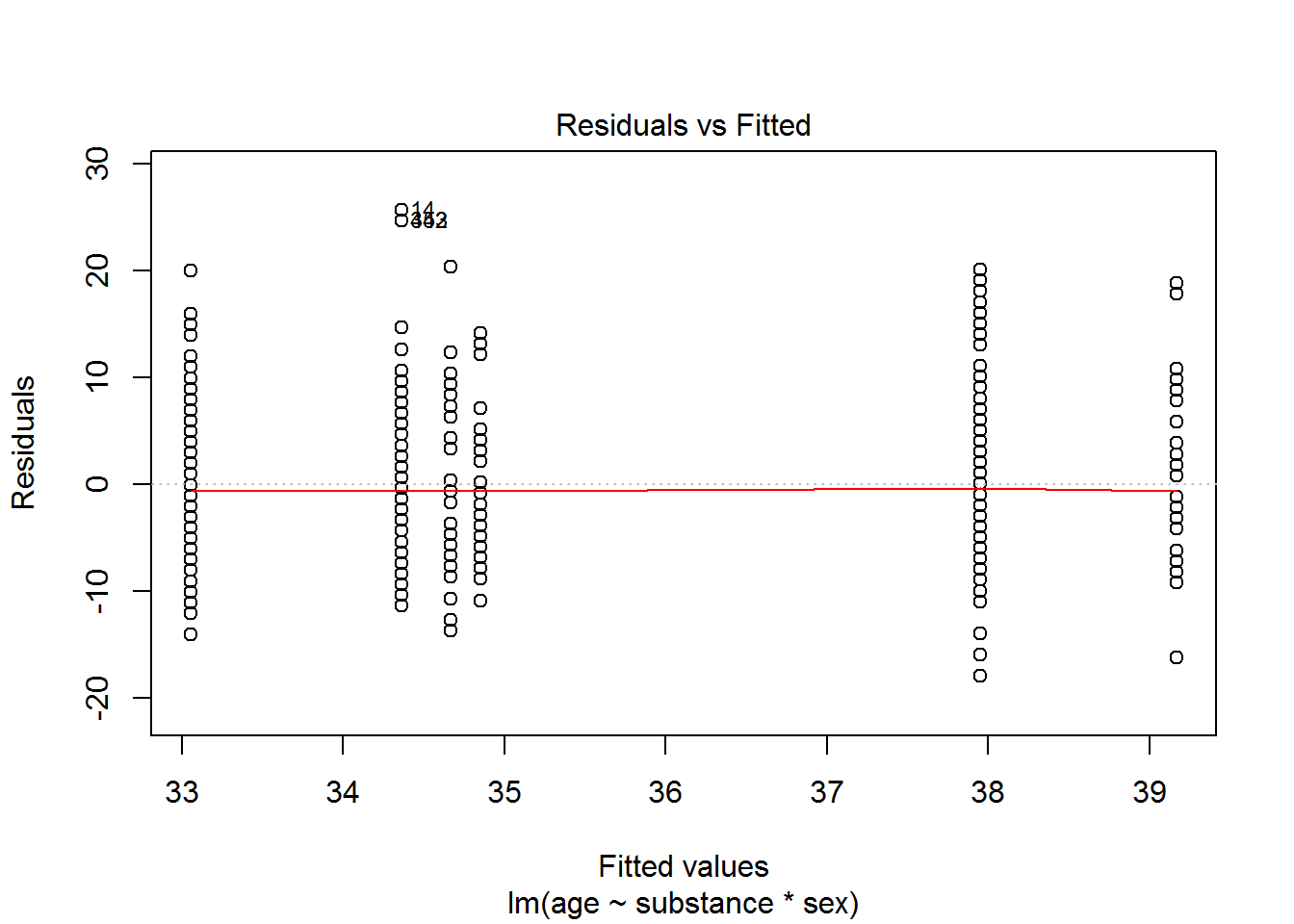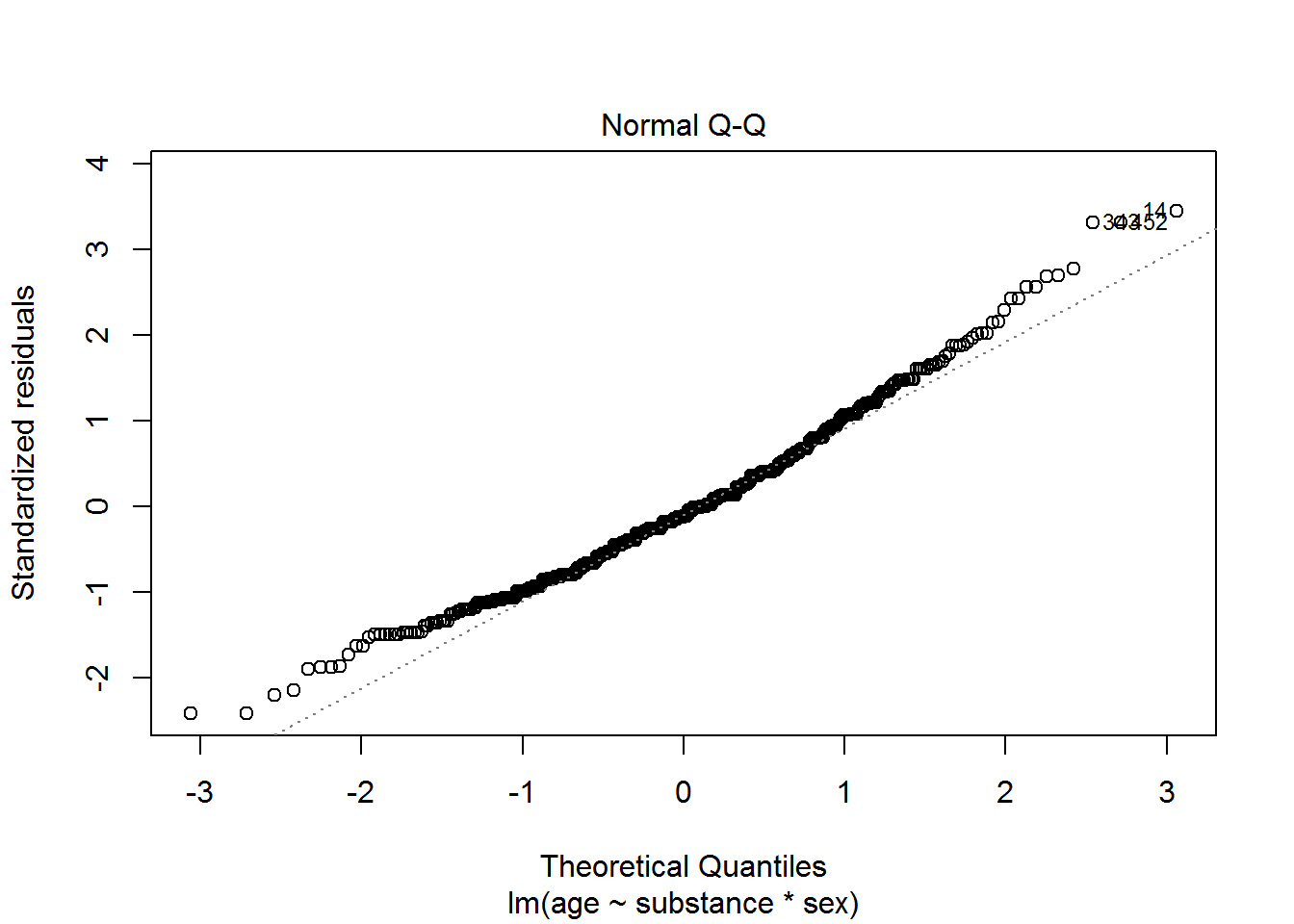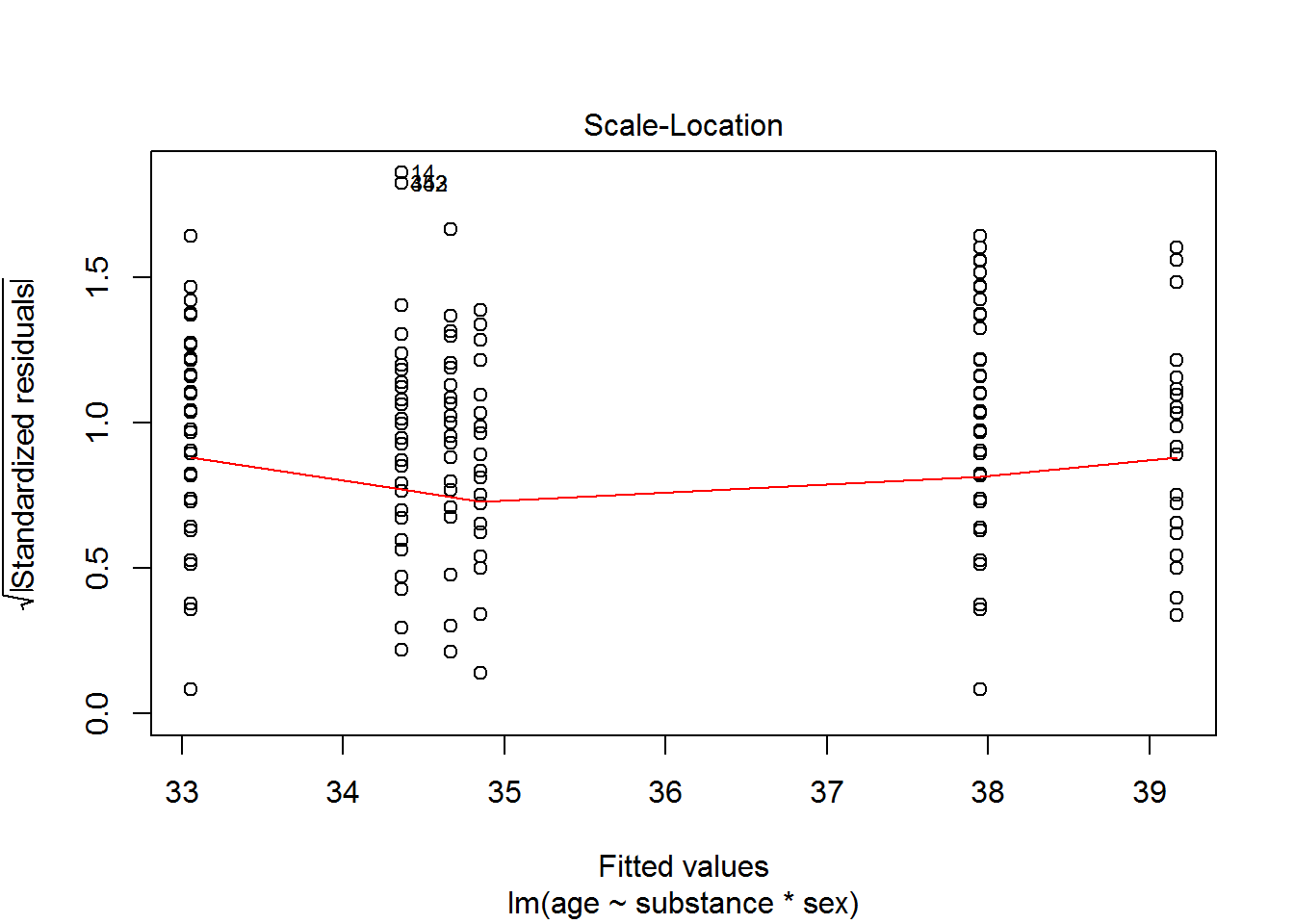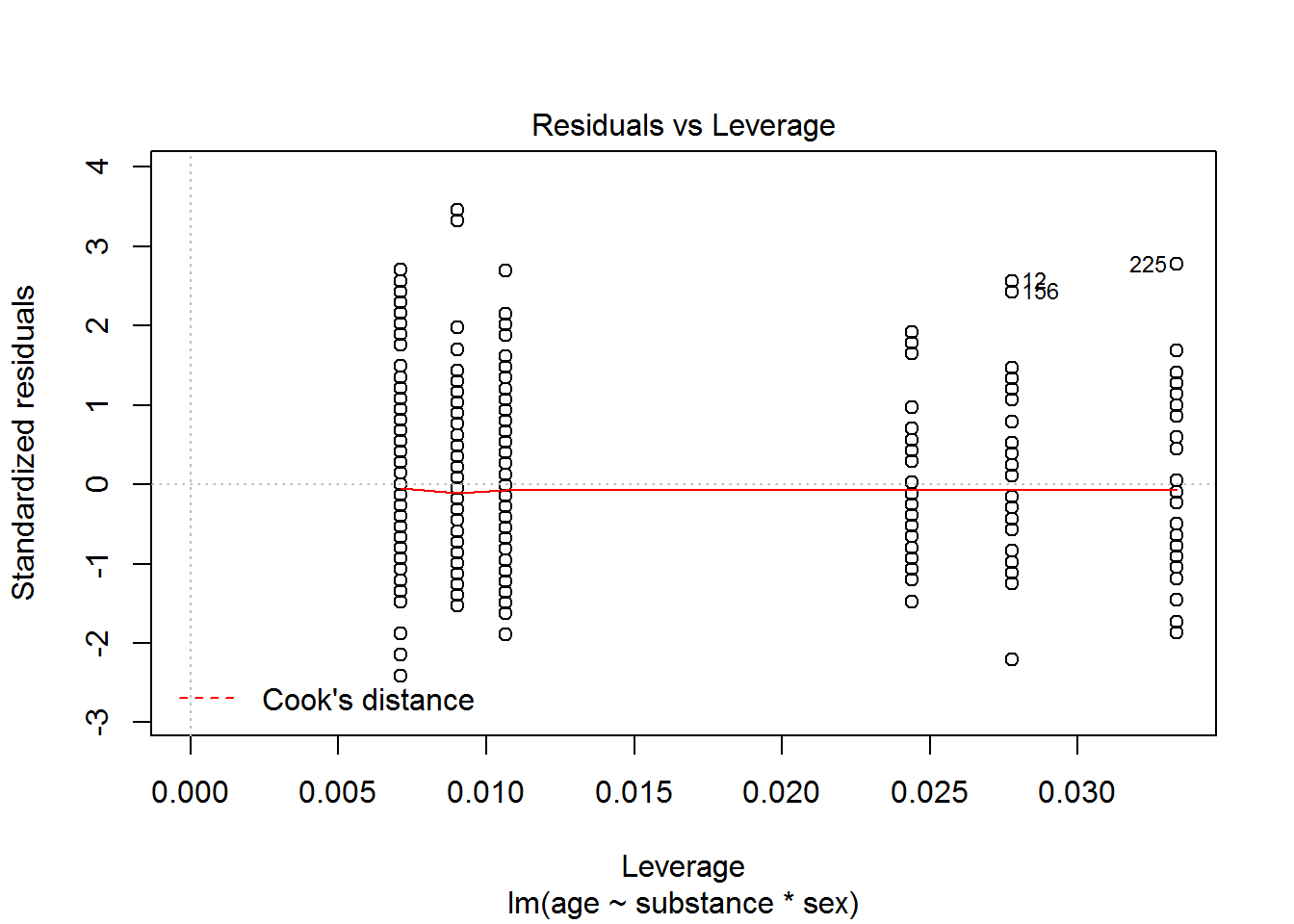## R Markdown

This is an R Markdown document. Markdown is a simple formatting syntax for authoring HTML, PDF, and MS Word documents. For more details on using R Markdown see http://rmarkdown.rstudio.com.

When you click the Knit button a document will be generated that includes both content as well as the output of any embedded R code chunks within the document. You can embed an R code chunk like this:

summary(cars)
##      speed           dist
##  Min.   : 4.0   Min.   :  2.00
##  1st Qu.:12.0   1st Qu.: 26.00
##  Median :15.0   Median : 36.00
##  Mean   :15.4   Mean   : 42.98
##  3rd Qu.:19.0   3rd Qu.: 56.00
##  Max.   :25.0   Max.   :120.00

## Including Plots

You can also embed plots, for example: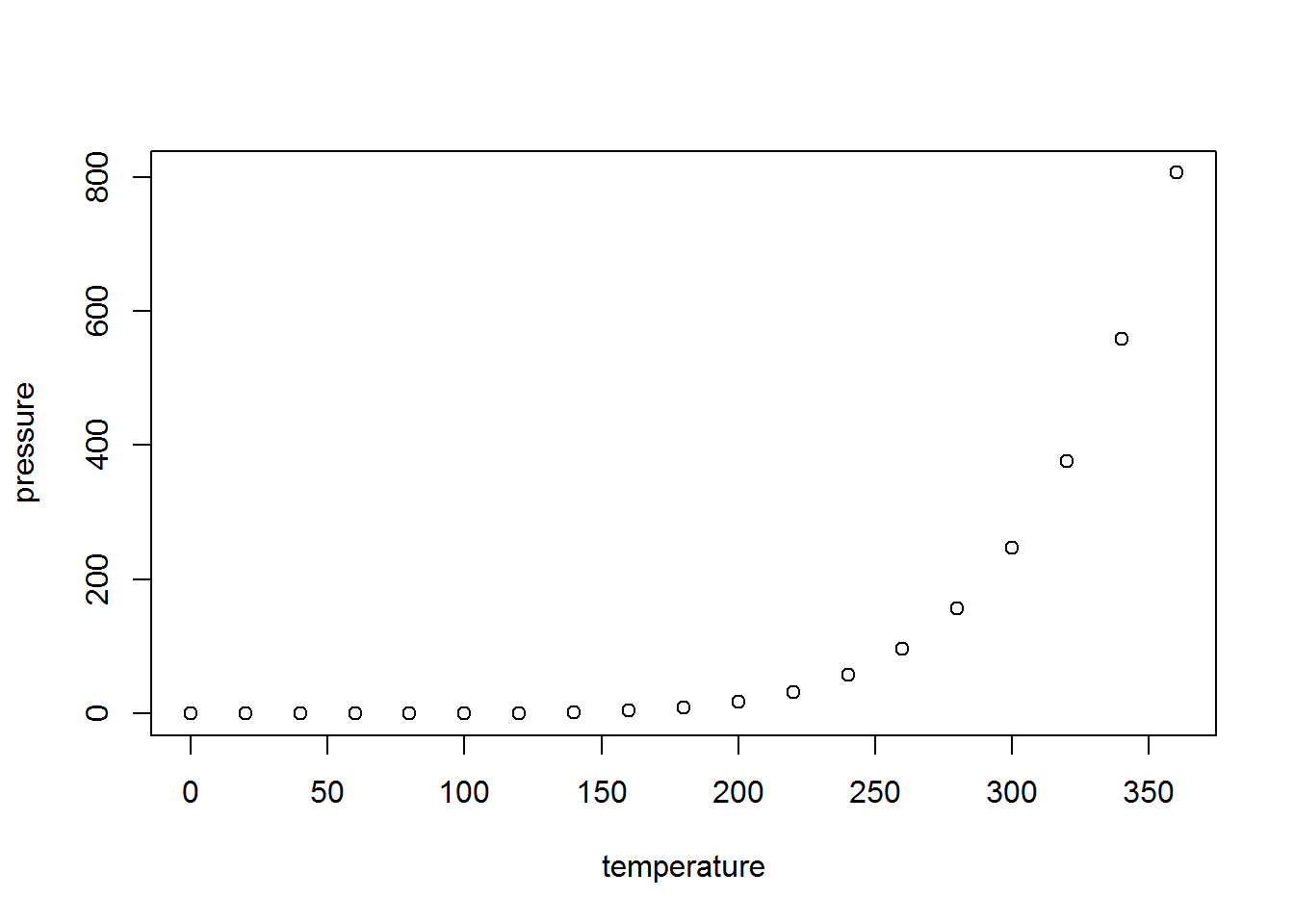Note that the echo = FALSE parameter was added to the code chunk to prevent printing of the R code that generated the plot.

### Text

Text can be decorated with bold or italics. It is also possible to

• include mathematics like $$e=mc^2$$ or $y = \beta_0 + \beta_1 x_1 + \beta_2 x_2$

# The most important template is

• goal(y~x|z,data=mydata,groups=…)
• What you want R to do is Goal : This determines the function to use(favstat, mean, SD, lm)
• What must R know to do that: This determines the inputs to the function, must identify variables and data frame
• it produces single and multiple variable graphical summaries
• it produces single and multiple variable numerical summaries
• linear models
• y: is y-axis
• x: is x-axis variable
• z: is conditioning variable (seperate panels)
• groups: conditioning variable (overlaid graphs)

# Numerical Summaries one variable

favstats(~Sepal.Length, data=iris)
##  min  Q1 median  Q3 max     mean        sd   n missing
##  4.3 5.1    5.8 6.4 7.9 5.843333 0.8280661 150       0

# Numerical summaries two variables

favstats(age~substance|sex,data=HELPrct)
##              sex min Q1 median   Q3 max     mean       sd   n missing
## 1 alcohol.female  23 33   37.0 45.0  58 39.16667 7.980333  36       0
## 2 cocaine.female  24 31   34.0 38.0  49 34.85366 6.195002  41       0
## 3  heroin.female  21 29   34.0 39.0  55 34.66667 8.035839  30       0
## 4   alcohol.male  20 32   38.0 42.0  58 37.95035 7.575644 141       0
## 5   cocaine.male  23 30   33.0 37.0  60 34.36036 6.889772 111       0
## 6    heroin.male  19 27   32.5 39.0  53 33.05319 7.973568  94       0
## 7         female  21 31   35.0 40.5  58 36.25234 7.584858 107       0
## 8           male  19 30   35.0 40.0  60 35.46821 7.750110 346       0
mean(age~substance|sex,data=HELPrct,.format="table") #tabular form
##   substance    sex     mean
## 1   alcohol female 39.16667
## 2   alcohol   male 37.95035
## 3   cocaine female 34.85366
## 4   cocaine   male 34.36036
## 5    heroin female 34.66667
## 6    heroin   male 33.05319

# Numerical summaries: Tables

tally(sex~substance,data=HELPrct)
##         substance
## sex      alcohol cocaine heroin
##   female      36      41     30
##   male       141     111     94
summary(sex~substance,data=HELPrct)
##  Length   Class    Mode
##       3 formula    call

# Next replace summary name by plot name

bwplot(age~substance|sex,data=HELPrct, .format="table")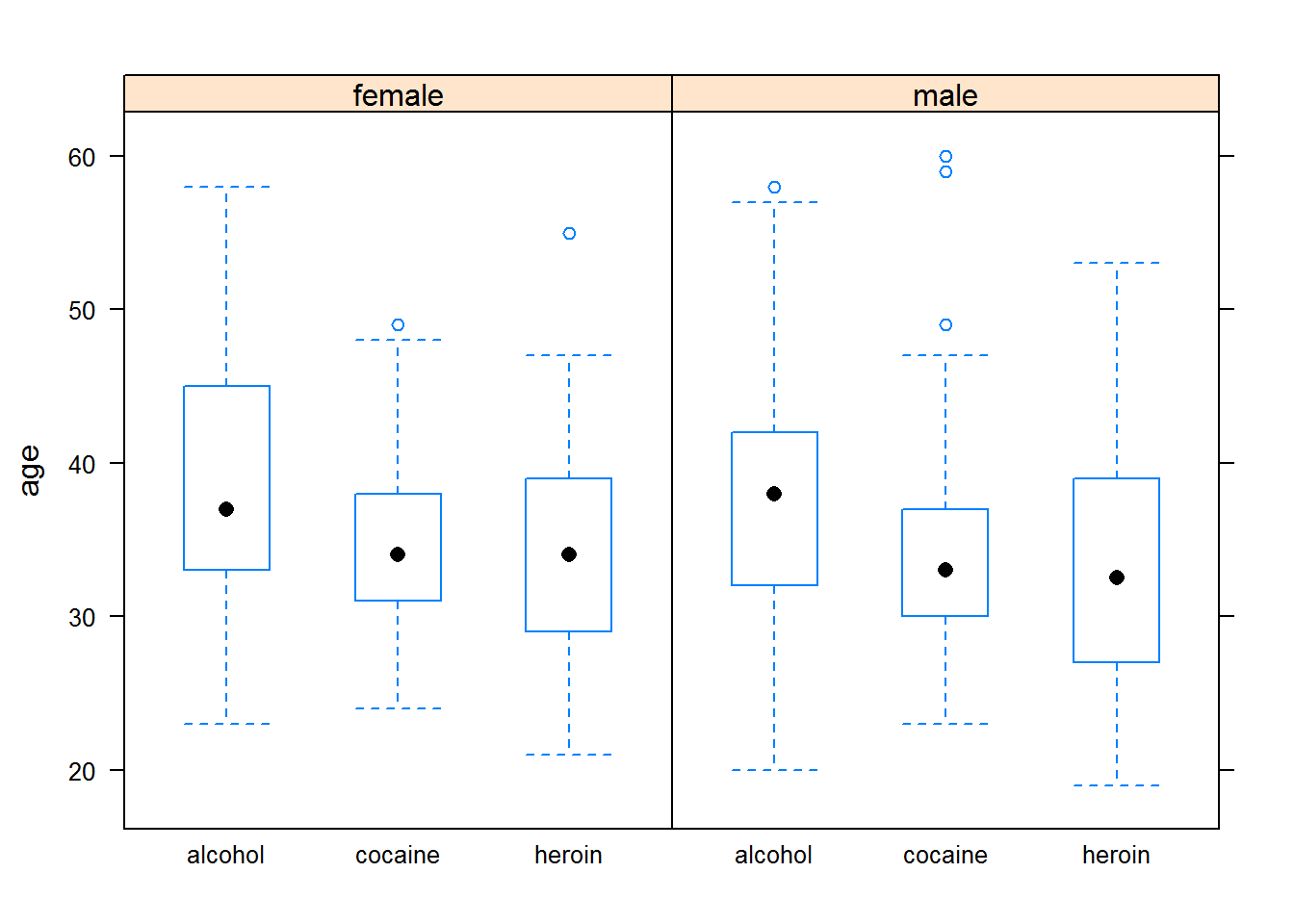# Graphical Summaries one variable

histogram(~age,data=HELPrct)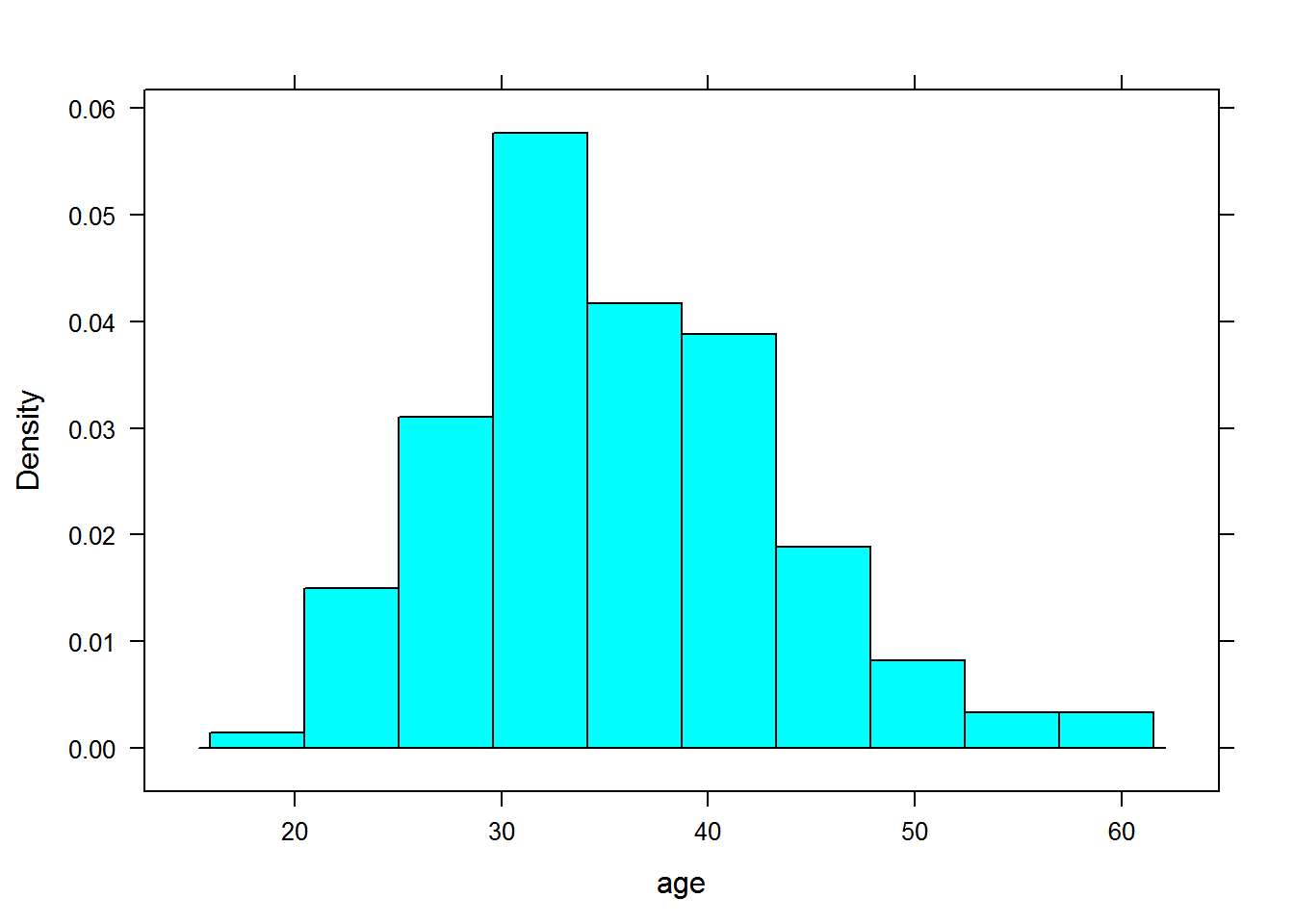densityplot(~age,data=HELPrct)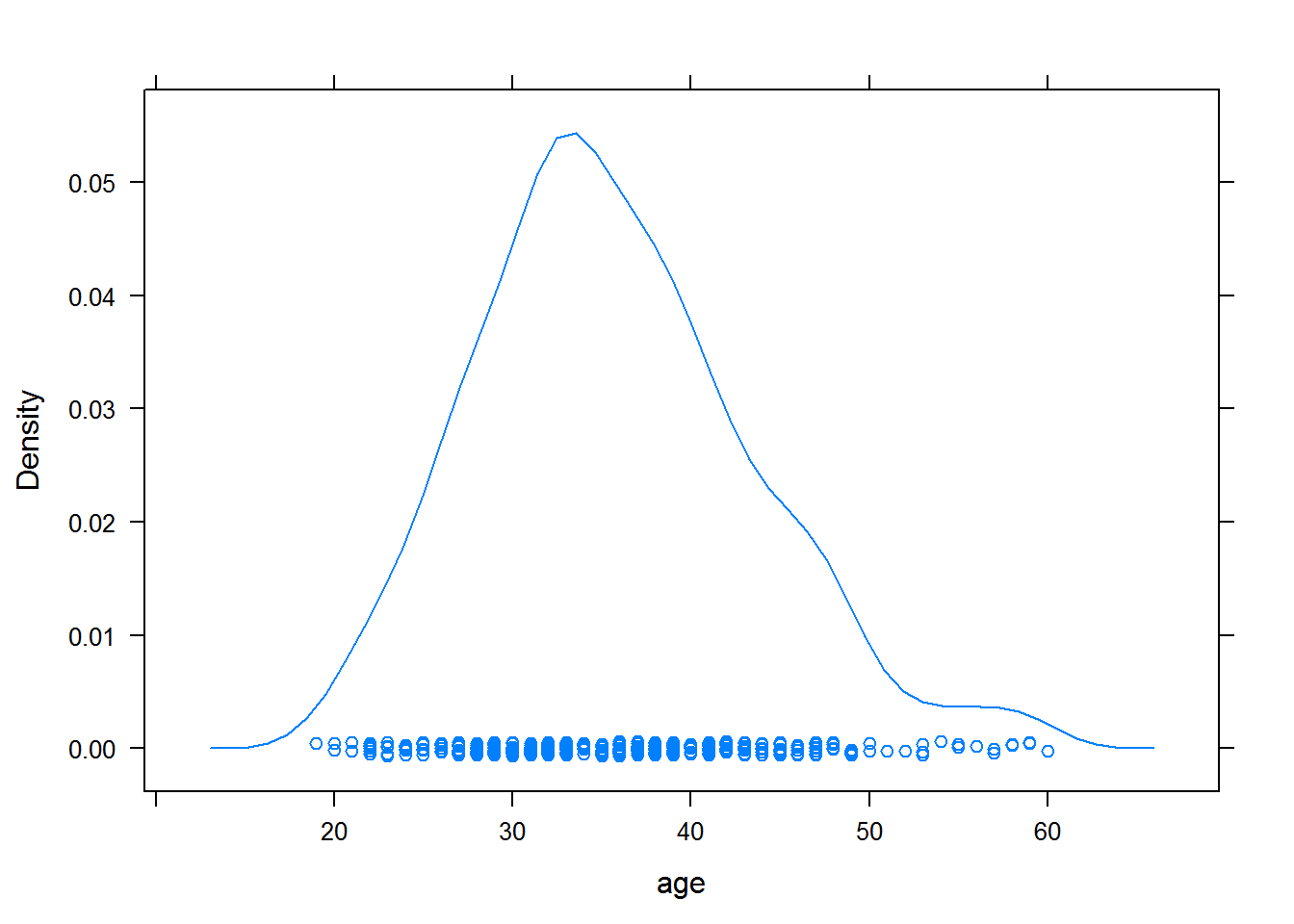bwplot(~age,data=HELPrct)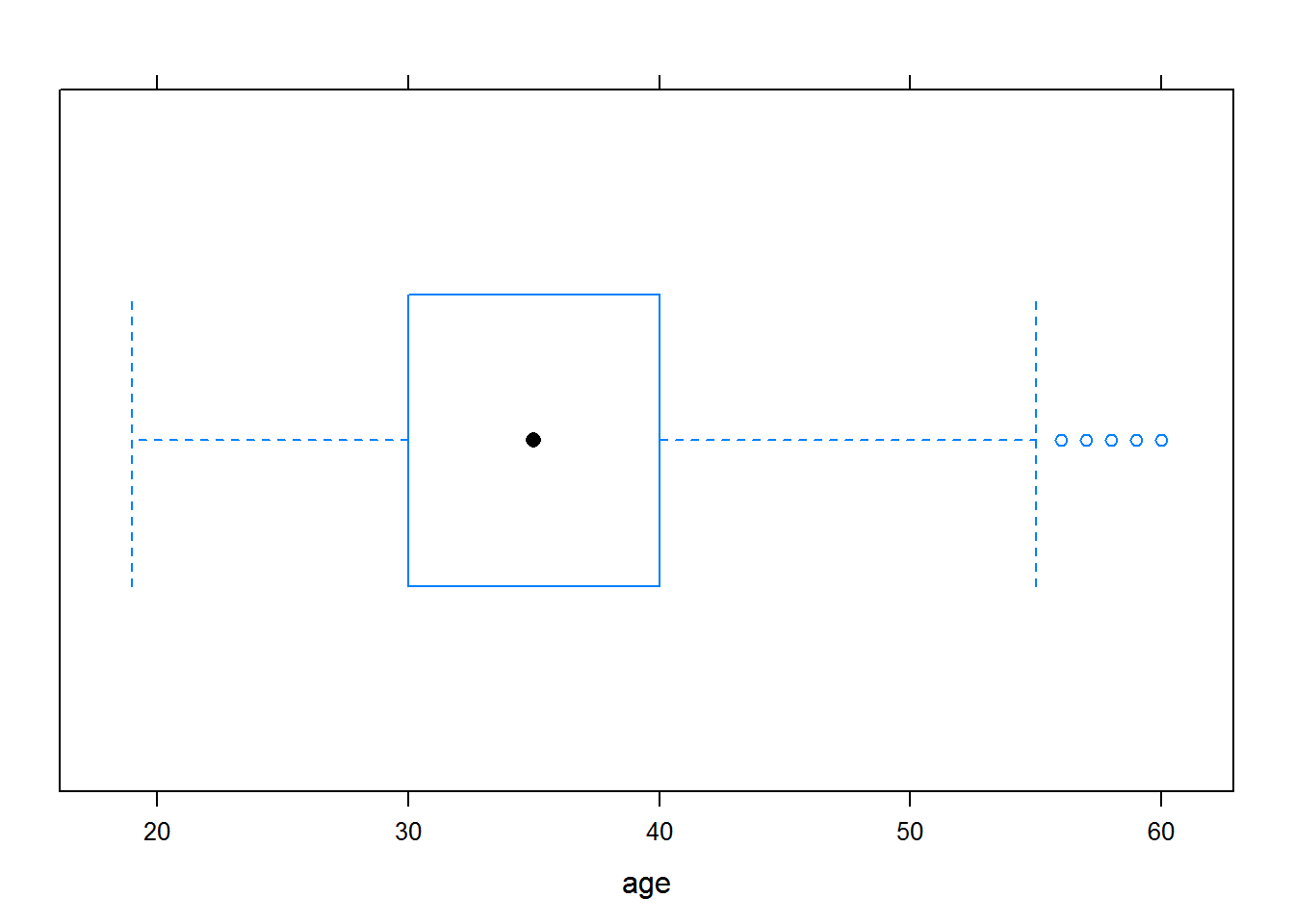qqmath(~age,data=HELPrct)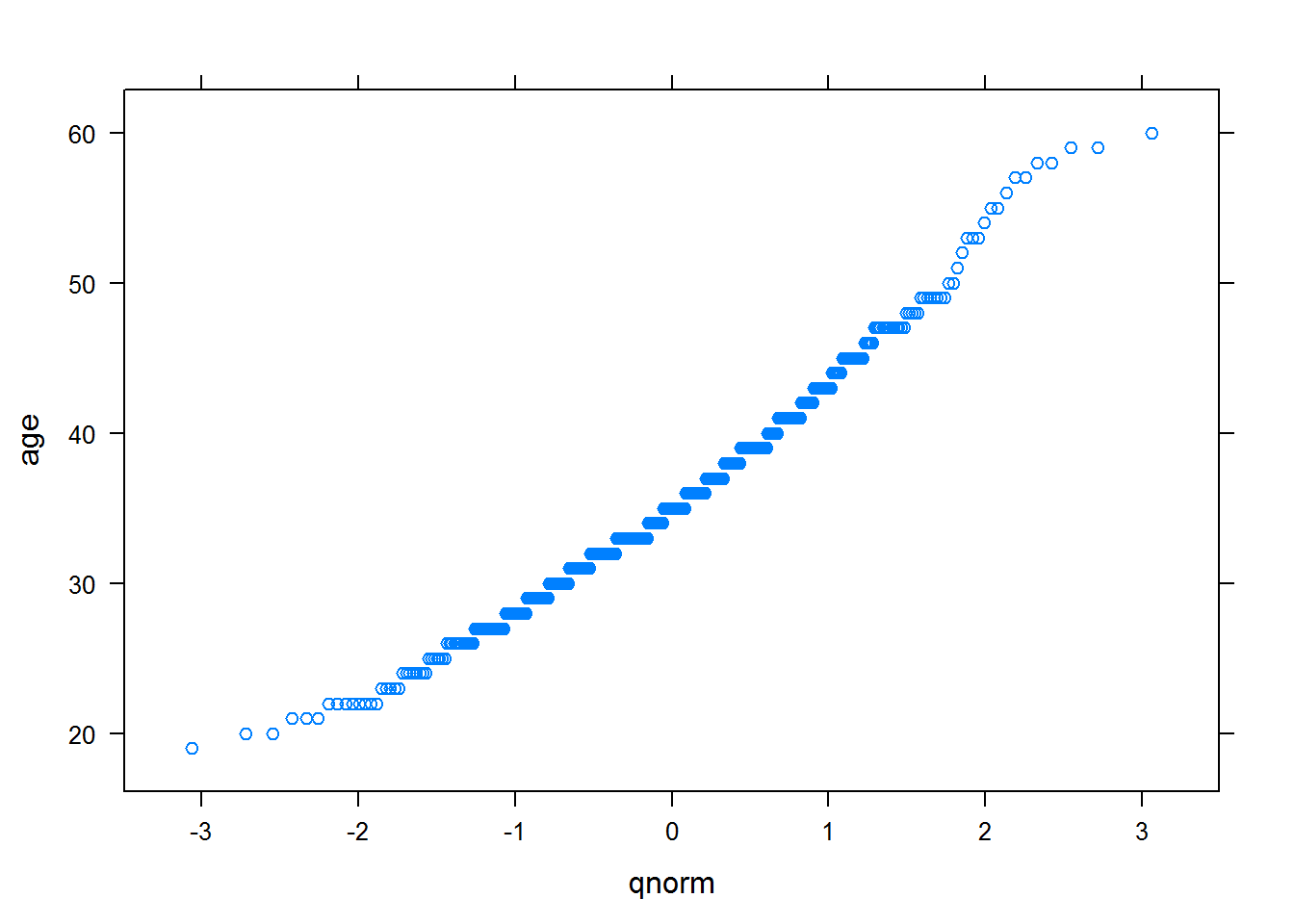freqpolygon(~age,data=HELPrct)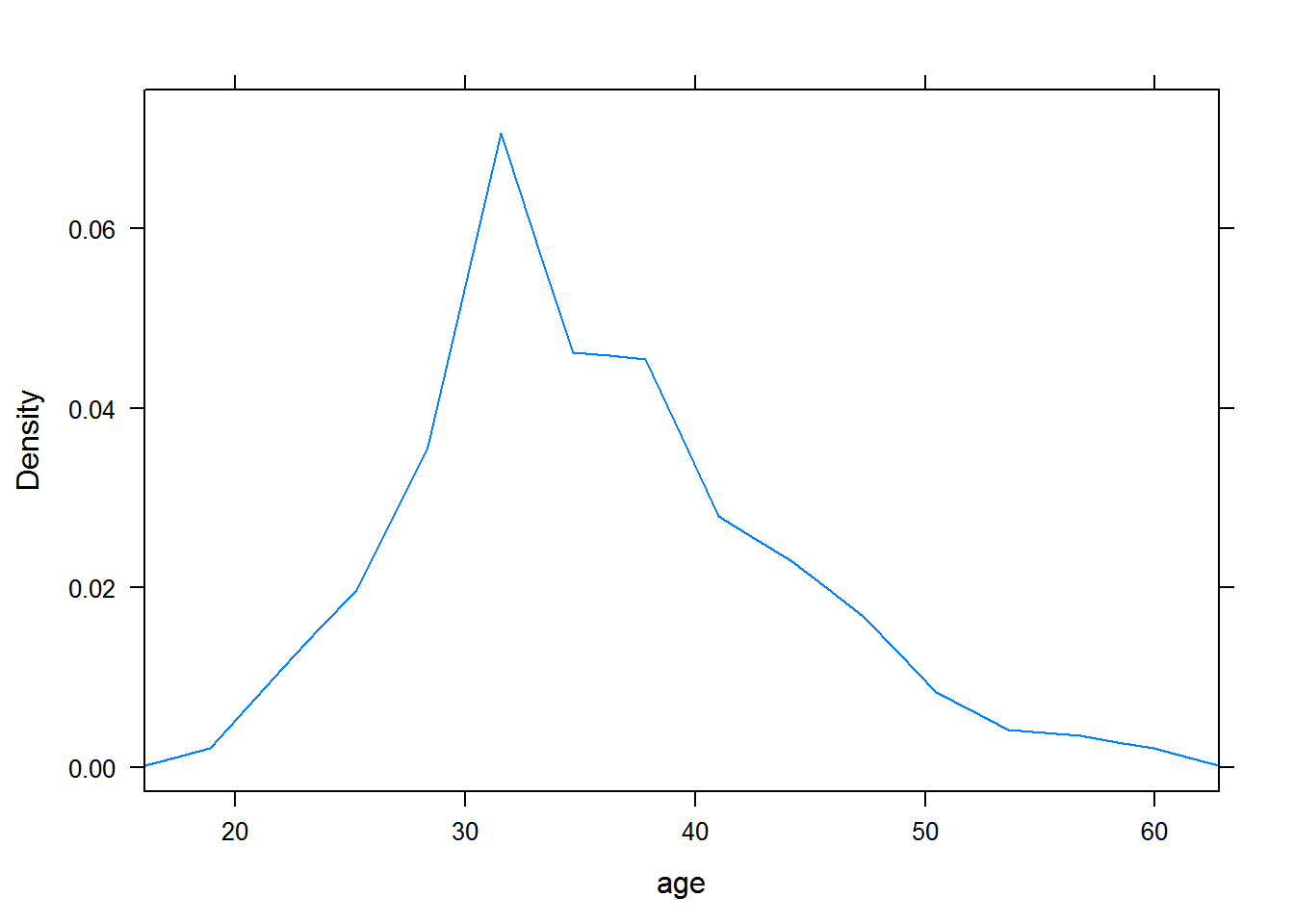bargraph(~age,data=HELPrct)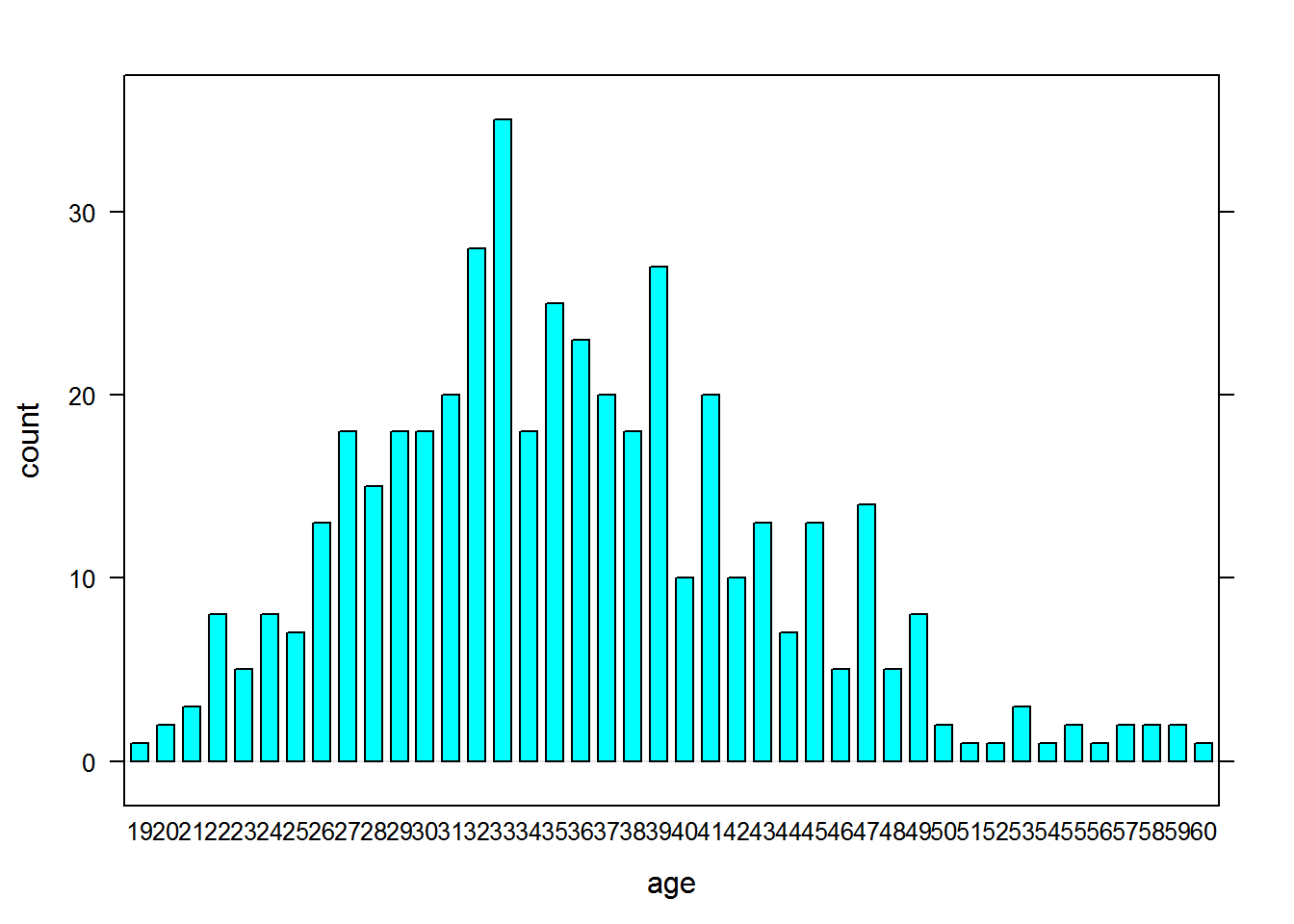# graphical summaries two variables * i1 average numebr of drinks consumed per day in past 30 days

xyplot(i1~age,data=HELPrct)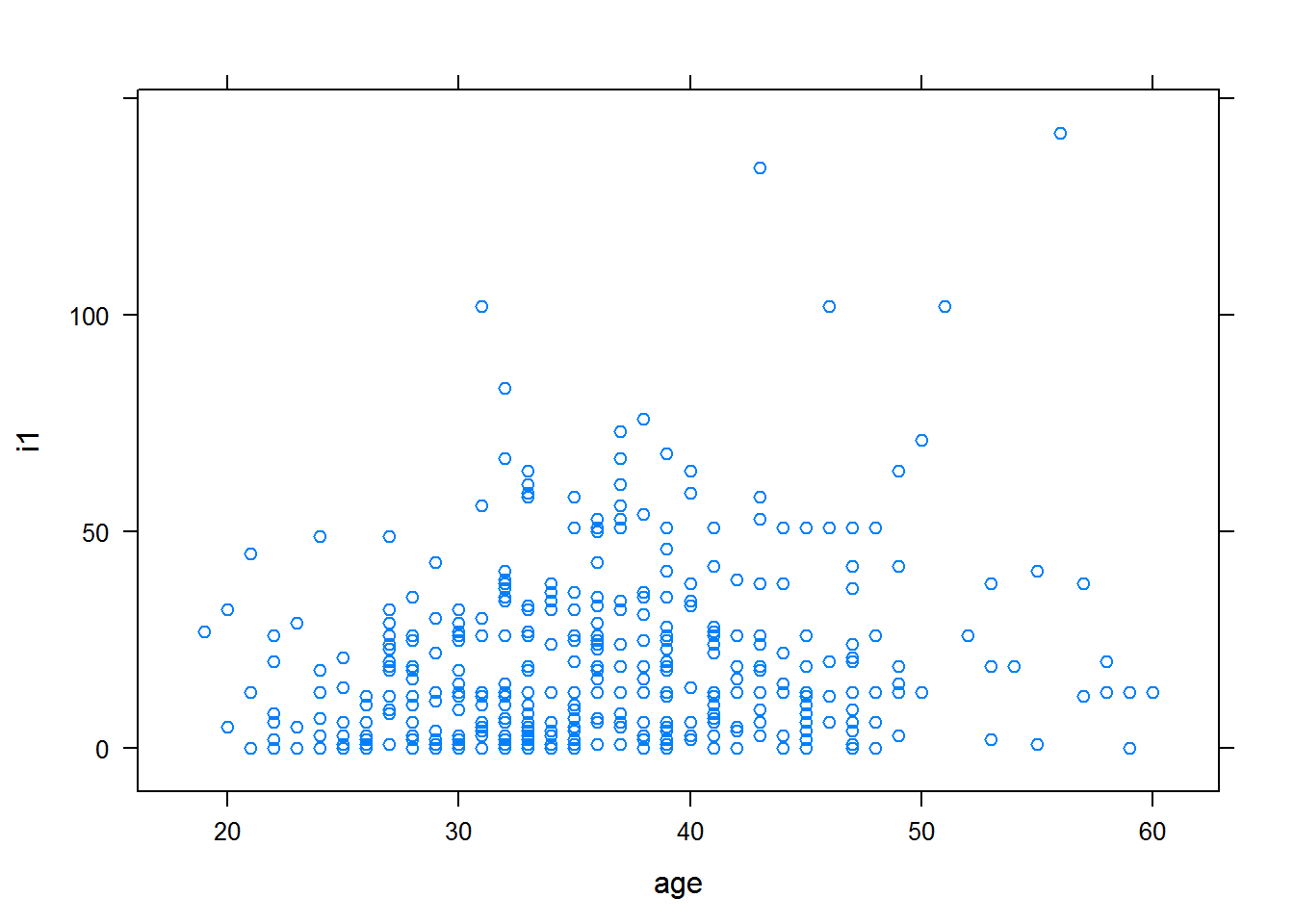bwplot(age~substance,data=HELPrct)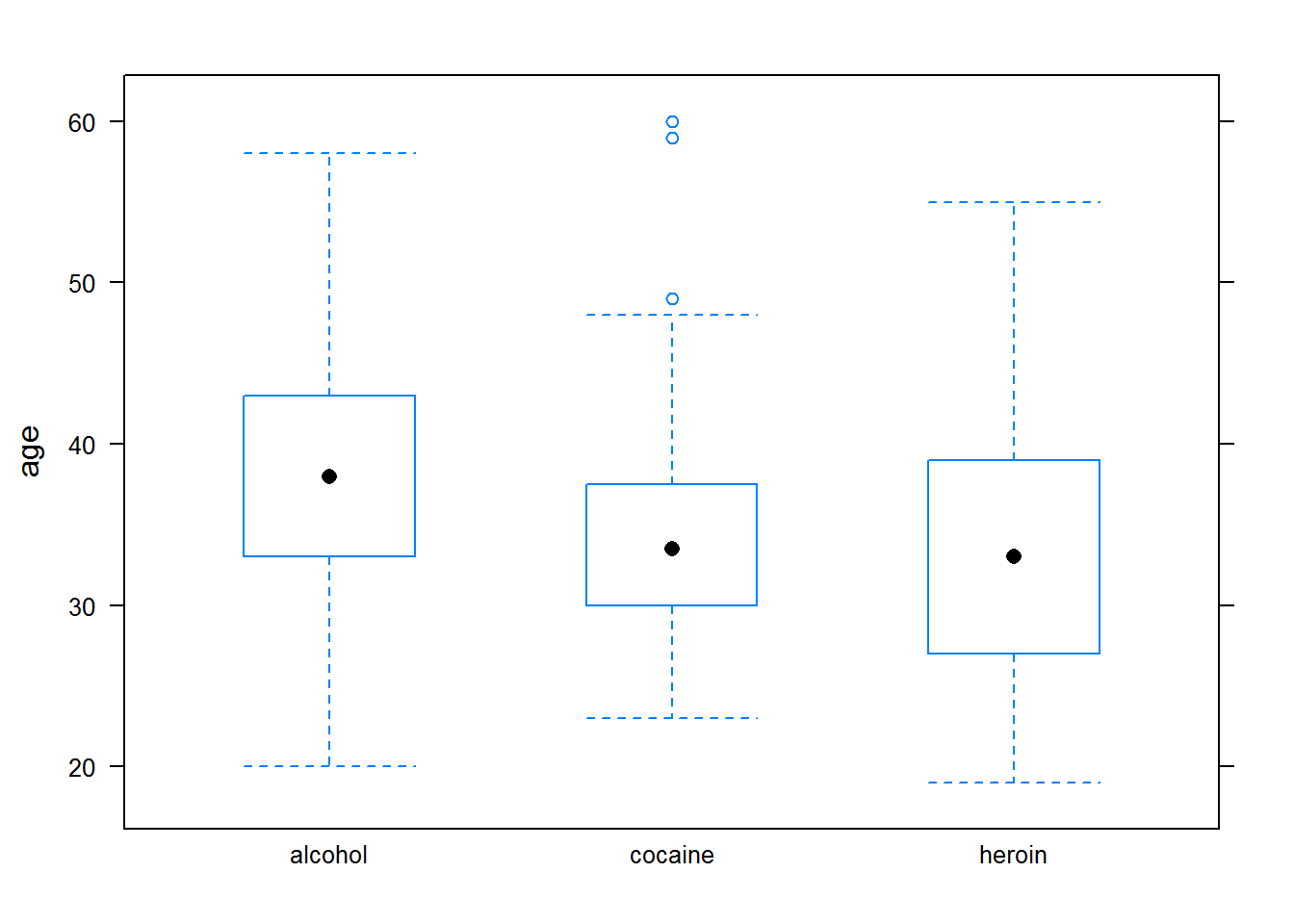# add groups = group to overlay * use y~x|z to create multipanel plots

densityplot(~age|sex,data=HELPrct,groups=substance, auto.key=TRUE)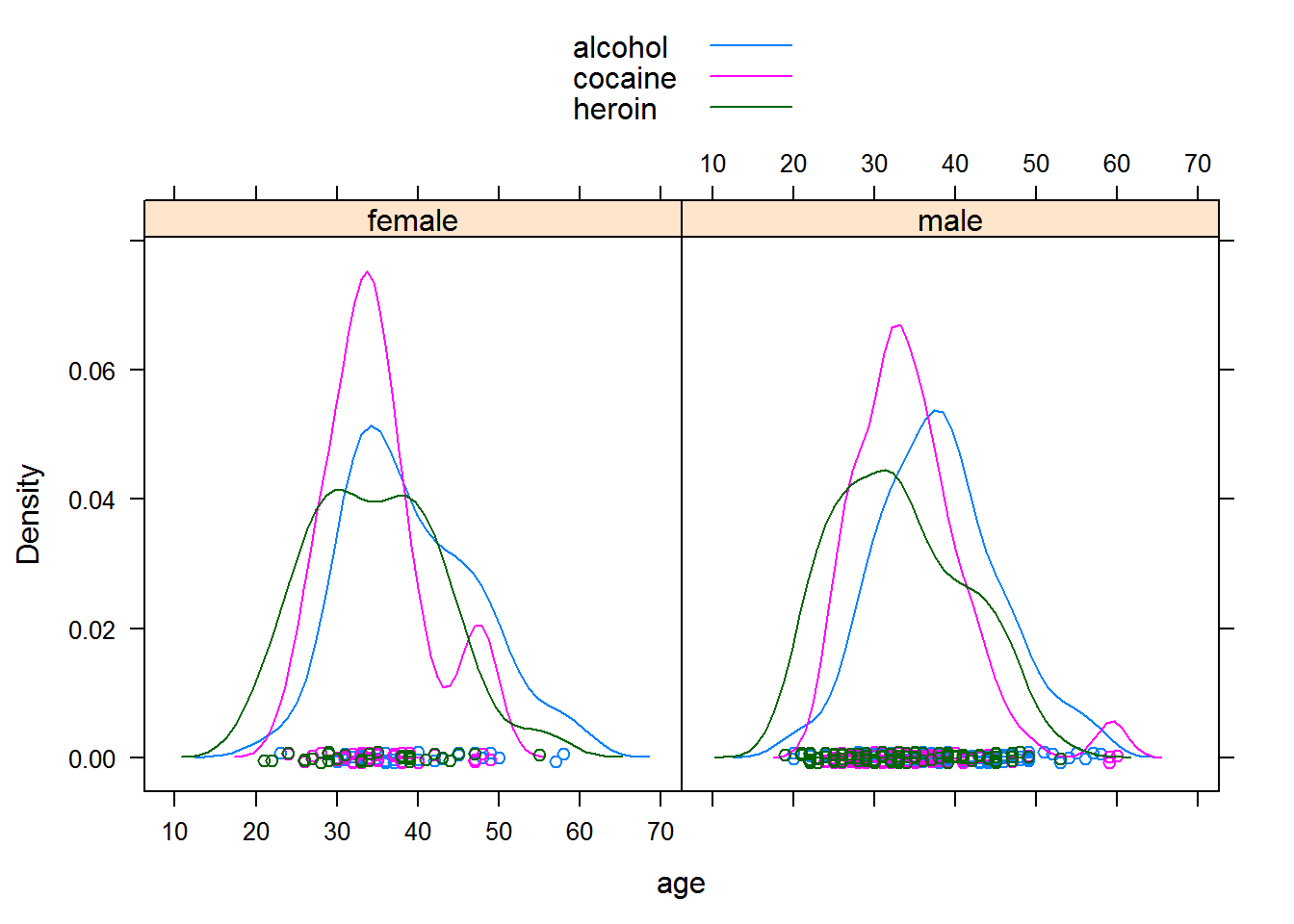• Create a fancy plot using kidsFeet data or faculty salary data
names(KidsFeet) # 4th graders feet
##  "name"       "birthmonth" "birthyear"  "length"     "width"
##  "sex"        "biggerfoot" "domhand"
#?KidsFeet

# some other things

• Mosaic package includes datasets, xtras: xchisq.test(data name),xpnorm(),mplot(),xqqmath
xpnorm( 700, mean=500, sd=100)
##
## If X ~ N(500, 100), then
##
##  P(X <= 700) = P(Z <= 2) = 0.9772499
##  P(X >  700) = P(Z >  2) = 0.02275013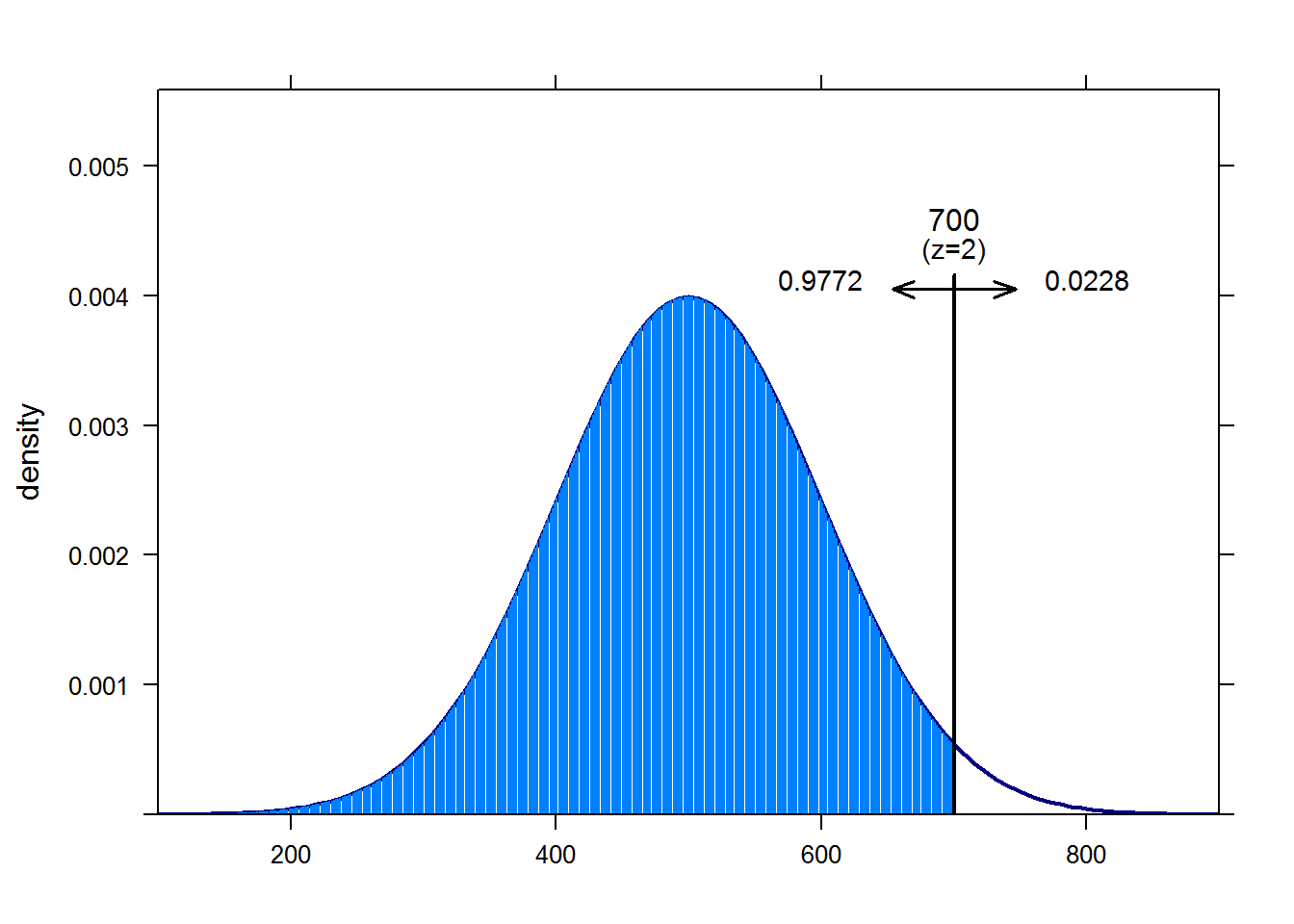##  0.9772499
xpnorm( c(300, 700), mean=500, sd=100)
##
## If X ~ N(500, 100), then
##
##  P(X <= 300) = P(Z <= -2) = 0.02275013
##      P(X <= 700) = P(Z <=  2) = 0.97724987
##  P(X >  300) = P(Z >  -2) = 0.97724987
##      P(X >  700) = P(Z >   2) = 0.02275013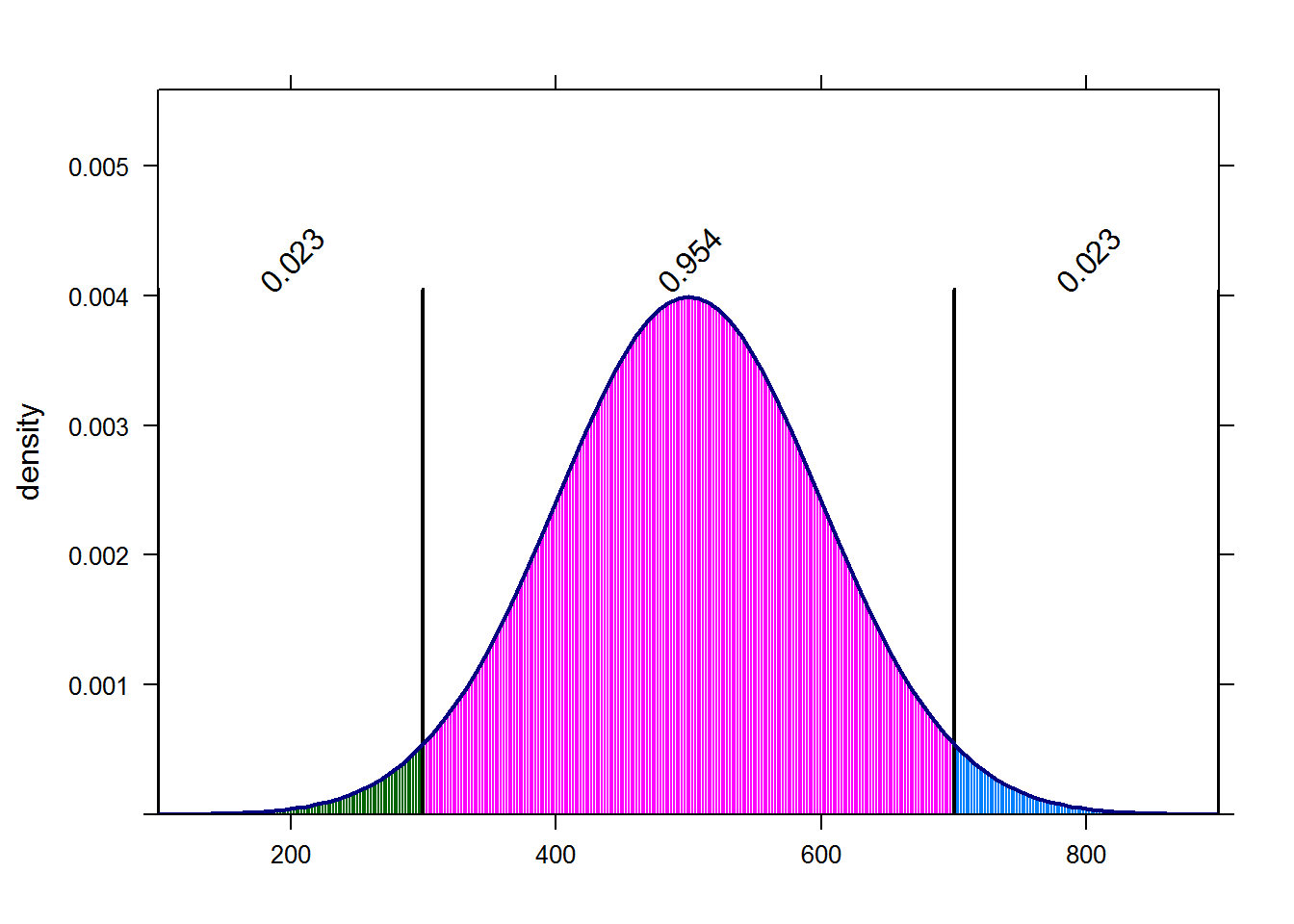##  0.02275013 0.97724987

# Modelling

• linear models lm(),glm() # linear models
a<-lm(age~substance*sex, data=HELPrct)
plot(a)